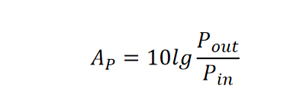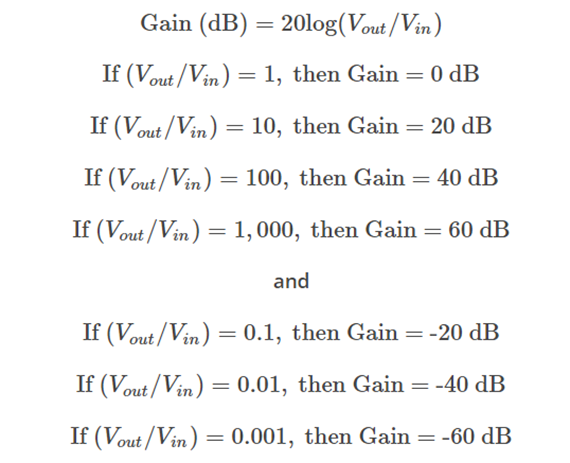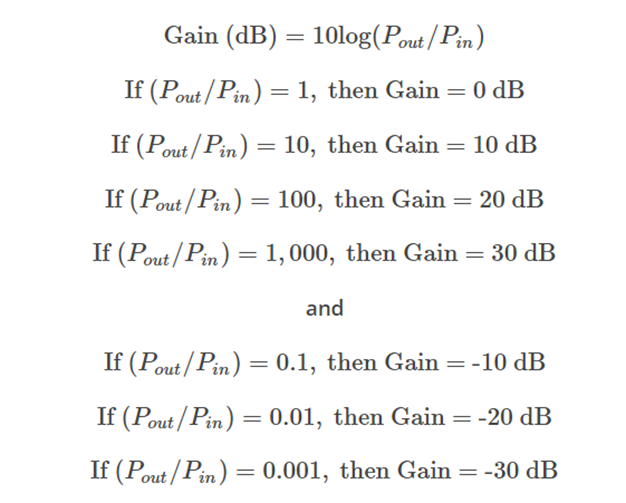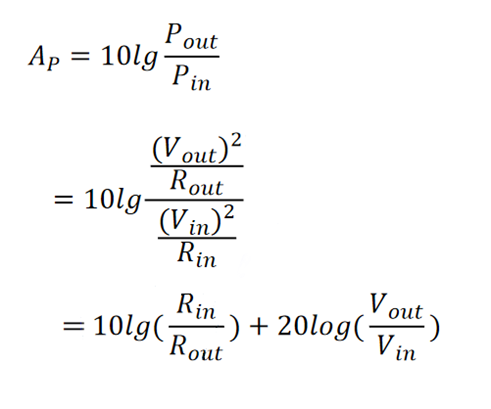1. Blog>
2. dB in Radio Frequency Design

# dB in Radio Frequency Design

by: Jul 21,2023 1000 Views 0 Comments Posted in Technology

When Bell, who invented the telephone, studied the sound, he defined the scale of the sound as bel. When the intensity of a sound increases tenfold, the increase in loudness is termed 1 bel. It is used as a unit for comparing power levels in electrical communication or sound intensity, corresponding to a ratio of 10 to 1. As the bel is a large number, its tenth part, the decibel (dB), was introduced: when the intensity of the sound increases by a factor of 1, the increase in loudness is 1 decibel. In other words, one-tenth of a bel is equal to one decibel. It is a unit used to measure the sound intensity or electrical signal power level by comparing it with a given level on a logarithmic scale.

On the decibel scale, the lowest audible sound (near complete silence) is 0 dB. A sound with 10 times the intensity is represented as 10 dB, while a sound with 100 times the intensity is 20 dB, and a sound with 1,000 times the intensity is 30 dB.

In RF (Radio Frequency) design, dB represents a logarithm with a base of 10, used to express ratios.Since dB is very convenient to use, does that mean we can freely apply it in all situations? For instance, when using a three-port power splitter as a power combiner, if we input 30 dBm signals into each of the two input ports, what will be the output power at the output port? Is it 30 dBm + 30 dBm = 60 dBm? The answer is incorrect. 3 dBm represents a big part of the power, so the correct answer is 30 dBm + 30 dBm = 33 dBm.

Apart from this, it's also important to pay attention to the distinction between voltage gain and power gain. The dB for voltage gain is 20 times the logarithm, with a base of 10, of the ratio between output voltage and input voltage.And the dB for power gain is 10 times the logarithm of the ratio between output power and input power.The reason for this phenomenon is that our primary focus is on radio frequency (RF) power. If we want to apply it to voltage, we need to start with the relationship between power and voltage:That is to say, power gain is the square of voltage gain, and this squaring operation results in the coefficient of 10 being multiplied by 2 in the logarithmic calculation.